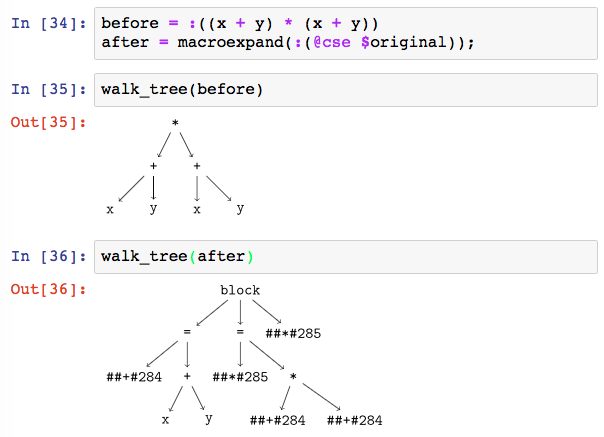16

3

4

2

# CommonSubexpressions.jl

This Julia package provides the `@cse` macro, which performs common subexpression elimination. That means that, given a piece of code like:

``````for i in 1:10
x[i] = foo(1) + i
end
``````

in which the function `foo(1)` is evaluated 10 times, the `@cse` macro will produce code that moves that expression out of the loop:

``````foo_1 = foo(1)
for i in 1:10
x[i] = foo_1 + i
end
``````

and thus only evaluates `foo(1)` once.

Arbitrarily complex nested expressions can be handled, and should result in more efficient code:

``````@cse inv(H) * (G + W) - (G + W)' * inv(H)
``````

becomes:

``````inv_H = inv(H)
G_plus_W = G + W
inv_H * G_plus_W - G_plus_W' * inv_H
``````

You can also wrap entire function definitions or code blocks:

``````@cse function foo(x)
[f(x) == i for i in 1:5]
end
``````

# Caveats

This package is very new and its results may not be correct. Please use it carefully and report any issues you find.

Any function called within a block wrapped in the `@cse` macro must be pure. That is to say, the function must have no side-effects. The `@cse` macro can not enforce or verify this. If your function has side-effects, then the common subexpression elimination may change the behavior of your program, since those side-effects will not happen as often as you had expected.

### Brief aside on function purity

A pure function is one with no side-effects. When we say that a function has side-effects, we mean that calling it somehow changes the state of your program, beyond just the value that it returns. A trivial function that does have a side-effect is:

``````f_counter = 0
function f(x)
global f_counter
f_counter += 1
2 * x
end
``````

which increases a counter `f_counter` every time it is called.

In addition, any function that mutates its input arguments can not be pure, since changing its input arguments constitutes a side effect.

# Visualization

The CSE transformation can be visualized using the TreeView.jl package. Here's a very simple example:# How it Works

This package does not (currently) construct a full data-flow graph like DataFlow.jl. Instead, it performs a few relatively simple steps:

1. Initialize the set of disqualified symbols to {}
2. Initialize the list of setup commands to []
3. Walk the expression tree, repeatedly performing these steps:
1. If an assignment operation (like `x = 5`) is encountered, then add the target of the assignment (`x` in this case) to the disqualified symbols.
2. If a function call is encountered and all the function arguments are either constants or symbols, and those symbols are not disqualified, then:
1. Replace the function call in the current expression with a newly generated symbol
2. Append to the setup commands an expression which performs the function call and assigns it to the new symbol
4. Return the transformed expression, with all the setup commands prepended.

This simple procedure ensures that we only cache functions whose inputs do not change within the given code block (assuming that all function calls are pure, as required above).

01/18/2017